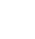# Random number generator in Excel

To generate a ‘Random’ set of numbers in Excel you just need to use the Randbetween formula as follows:

``` =Randbetween(1,5000) ```

This will put a random number between 1 and 5000 in the cell where the formula is. Drag this down to as many cells as you like.